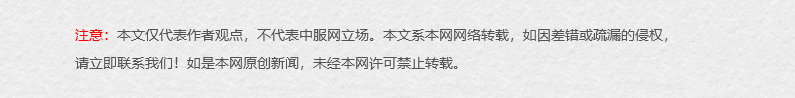该系列短片共分为5集，介绍了ECONYL尼龙纱原材料的不同回收途径，让观众一睹制造这一开创性面料的工厂设施内部真容，进一步了解这一倡议的组成内容。

正如片名所示，影片的重点是每个个体的人，是我们自己—我们每个人的举动，或正面或负面，都会影响到这个星球的承载能力。个人行为会造成全球性影响，每个人，每件事，都会带来改变。

ECONYL再生尼龙的原材料为废弃塑料，回收自全球各地的填埋场和海洋。### 热点专题

•上海休闲装招商
•上海内衣招商
•上海户外服装招商
•上海服饰招商
•上海欧美女装招商
•上海男装代理
•上海男装招商
•上海衣服招商
•上海运动装招商
•中山内衣招商
•中山婴幼装招商
•中山服装招商
•厦门休闲装招商
•厦门牛仔装招商
•浙江休闲装加盟

### 热榜

• 招商
• 专题
• 品牌
• 地区
• ECA
• 梵凯
• 艺梦来
• 第二印象
• 皇后婴儿
• RBIGX
• 唐束
• 冰轩
• 格蕾斯
• 兰缔吉儿
• 的纯
• 圣天虹
• 约布
• 纯粹
• 适时雅集
• 金果果
• 博铂定制
• 梵思诺
• 大嘉汇女装批发商城
• 拉斐贝贝
• 辛芭狗
• 木子
• EIZSA
• 艾卓拉
• 迪图
• 尼赫菲
• 秦艺
• 秦艺
• 尚来
• 尚来
• 狄梵思黛
• 迪柯顿
• JOJO
• 林枝梅
• 爱为
• 都市衣柜
• 都市新感觉
• 适时雅集
• 衣佰芬
• 芙丽芙丽
• 红贝缇
• 卡索
• 例格
• 曼妮芬
• 牧柔
• 夺宝奇兵
• 唯简
• 迷你品
• 小米米
• 米欧米
• 米尔罗
• 木子
• 茜子
• 荣霞世家
• 圣芭黎
• 诗萌
• 饰典
• 松鹰
• 头彩
• 薇欧拉
• DOTACOKO
• 适时雅集
• 吉米赛欧
• T&W
• 颜诺国际
• 罗蒙
• 丽比多
• poipoilu
• 乔帛
• 韩菲斯
• 伊布都
• 卡度尼
• 桃花季
• D2M
• DerliGalam
• 曼妮芬
• 牧柔
• 旺华
• 唯简
• 中纺标
• 金果果
• 小积木
• 底色
• 诺奇
• 袋鼠
• 唯简尚
• LIKEBAG
• 美亚展示
• ANOTHER ONE
• TW
• 福州服装
• 福建服装
• 合肥服装
• 安徽服装
• 丽水服装
• 台州服装
• 金华服装
• 绍兴服装
• 湖州服装
• 嘉兴服装
• 温州服装
• 宁波服装
• 杭州服装
• 浙江服装
• 扬州服装
• 盐城服装
• 南通服装
• 苏州服装
• 常州服装
• 无锡服装
• 南京服装